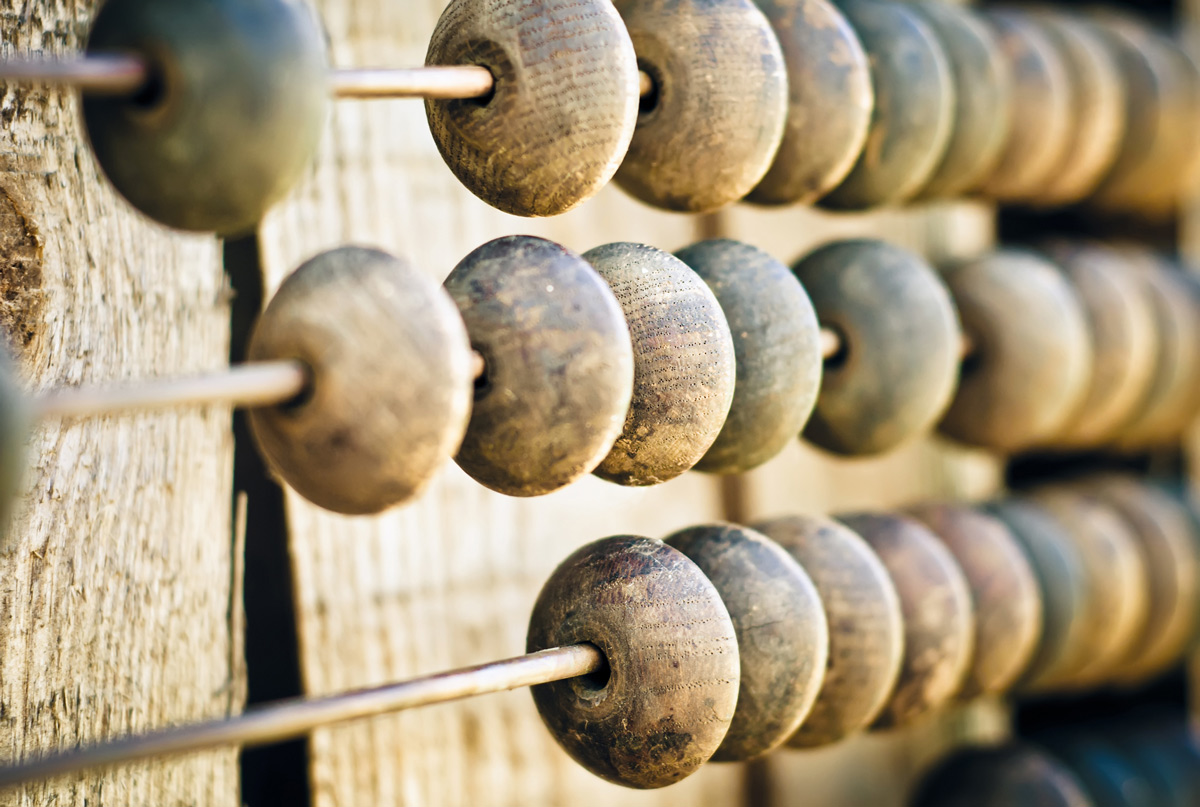# Cool Math FactsThe science of Math may be one of the more demanding subjects to learn, but it is also a world full of strange facts and discoveries. Here are some cool and amazing facts about Math complements of C. Bhaskar, Professor of Mathematics. Take a look and see if you recognize any of these truisms:

Notches (cuts or indentations) on animal bones prove that humans have been doing mathematics since around 30,000 BC.

What comes after a million, billion, and trillion? A quadrillion, quintillion, sextillion, septillion, octillion, nonillion, decillion and undecillion.

40 when written “forty” is the only number with letters in alphabetical order, while “one” is the only one with letters in reverse order.

Zero (0) is the only number that cannot be represented by Roman numerals.Do you know the magic of nine? Multiply any number with nine and then sum all individual digits of the result (product) to make it single digit. The sum of all these individual digits will always be nine.The abacus is considered to be the origin of the calculator.

Have you ever noticed that the opposite sides of a die always add up to seve12,345,678,987,654,321 is the product of 111,111,111 x 111,111,111. Notice the sequence of the numbers 1 to 9 and back to 1.

If you add up the numbers 1-100 consecutively (1+2+3+4+5…) the total is 5050.

2 and 5 are the only primes that end in 2 or 5.

From 0 to 1,000, the letter “A” only appears once: one thousand.

If there are 50 students in a class then it’s virtually certain that two will share the same birthday.

Among all shapes with the same perimeter, a circle has the largest area.

Among all shapes with the same area, a circle has the shortest perimeter.

An icosagon is a shape with 20 sides.While working out mathematical equations, the Greek mathematician Pythagoras used little rocks to represent numbers. Hence the name Calculus was born which means pebbles in Greek.

Have you heard about Fibonacci? It is the sequence of numbers wherein a number is the result of adding the two numbers before it. Here is an example: 1, 1, 2, 3, 5, 8, 13, 21, 34, and so on.

Want to remember the value of Pi (3.1415926) in an easy way? You can do it by counting each word’s letters in “May I have a large container of coffee?”

Have you heard about a Palindrome Number? It is a number that reads the same backwards and forward, e.g. 12421.So you see, there is no reason to let Math get the best of you. Just share one of its odd, amazing facts and you will dominate the field!